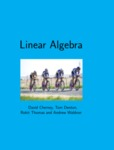## Open Access TextbooksLinear Algebra

#### Contributors

David Cherney - Author / Tom Denton - Author / Andrew K. Waldon - Author

#### Description

We believe the entire book can be taught in twenty five 50-minute lectures to a sophomore audience that has been exposed to a one year calculus course. Vector calculus is useful/ but not necessary preparation for this book/ which attempts to be self-contained. Key concepts are presented multiple times/ throughout the book/ often first in a more intuitive setting/ and then again in a definition/ theorem/ proof style later on. We do not aim for students to become agile mathematical proof writers/ but we do expect them to be able to show and explain why key results hold. We also often use the review exercises to let students discover key results for themselves; before they are presented again in detail later in the book. The book has been written such that instructors can reorder the chapters (using the La- TeX source) in any (reasonable) order and still have a consistent text. We hammer the notions of abstract vectors and linear transformations hard and early/ while at the same time giving students the basic matrix skills necessary to perform computations. Gaussian elimination is followed directly by an “exploration chapter” on the simplex algorithm to open students minds to problems beyond standard linear systems ones. Vectors in Rn and general vector spaces are presented back to back so that students are not stranded with the idea that vectors are just ordered lists of numbers. To this end/ we also labor the notion of all functions from a set to the real numbers. In the same vein linear transformations and matrices are presented hand in hand. Once students see that a linear map is specified by its action on a limited set of inputs/ they can already understand what a basis is. All the while students are studying linear systems and their solution sets/ so after matrices determinants are introduced. This material can proceed rapidly since elementary matrices were already introduced with Gaussian elimination. Only then is a careful discussion of spans/ linear independence and dimension given to ready students for a thorough treatment of eigenvectors and diagonalization. The dimension formula therefore appears quite late/ since we prefer not to elevate rote computations of column and row spaces to a pedestal. The book ends with applications–least squares and singular values. These are a fun way to end any lecture course. It would also be quite easy to spend any extra time on systems of differential equations and simple Fourier transform problems.

#### Subject 1

Mathematics - Pure

#### Subject 2

Mathematics - Pure

#### Publisher

University of California/ Davis

#### Resources

Open Textbook Library